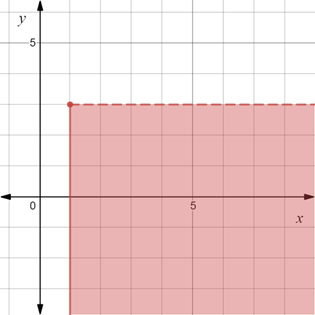# To graph : The region provided by the set { ( x , y ) | x ≥ 1 and y &lt; 3 } .### Precalculus: Mathematics for Calcu...

6th Edition
Stewart + 5 others
Publisher: Cengage Learning
ISBN: 9780840068071### Precalculus: Mathematics for Calcu...

6th Edition
Stewart + 5 others
Publisher: Cengage Learning
ISBN: 9780840068071

#### Solutions

Chapter 1.8, Problem 31E
To determine

## To graph: The region provided by the set {(x,y)|x≥1 and y<3} .

Expert Solution

### Explanation of Solution

Given information:

The set, {(x,y)|x1 and y<3} .

Graph:

The graph of the region provided by the set {(x,y)|x1 and y<3} is sketched in the coordinate plane.

Construct broken horizontal line y=3 and solid vertical line x=1 .

Shaded the region right of the line x=1 and below the line y=3 .

The region obtained is provided below,Interpretation:

The region provided by the set {(x,y)|x1 and y<3} is shaded region. The solid vertical line at x=1 denote that they are included in the region.The broken horizontal line at y=3 denotes that it is not included in the region. The region consists of all those points whose y-coordinate is less than 3 and x-coordinate is greater than or equal to 1. Therefore, shaded region is the region to the right of x=1 and below y=3 .

### Have a homework question?

Subscribe to bartleby learn! Ask subject matter experts 30 homework questions each month. Plus, you’ll have access to millions of step-by-step textbook answers!